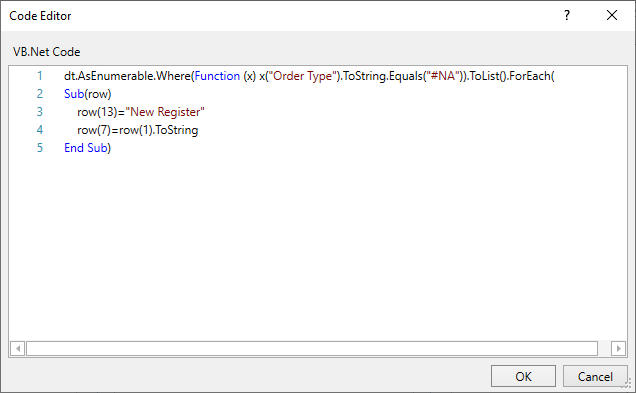# How to use Linq?

I want to read excel If column Order Type = #N/A
write text = New register In Column N
and copy data from Col B → write in column H

I think if use linq , It fastest than for each row.

@Maria99

There are two operations involved → writing New Register text and and copying column value
Both the task are to be done only when Order Type = #NA ?

Can you share the sample excel file.

You can try the following methodThe Code used is

``````dt.AsEnumerable.Where(Function (x) x("Order Type").ToString.Equals("#NA")).ToList().ForEach(
Sub(row)
row(13)="New Register"
row(7)=row(1).ToString
End Sub)
``````

Finally you can write the data table (`inputDT`) to the excel file using the Write Range Activity

Change Cell Value.xaml (5.0 KB)

1 Like

@kumar.varun2 I use follow you suggest.
But after run rows #N/A is blank (column H and I)

Find attached about input as below:
data.xlsx (45.3 KB)

Please guide me for solve it.

Hi @Maria99

Try with the code below

``````dt.AsEnumerable.Where(Function (x) String.IsNullOrEmpty(x("Order Type").ToString.Trim)).ToList().ForEach(
Sub(row)
row("Order Type")="New Register"
row("Order Date")=row("Activation Date").ToString
End Sub)
``````

Attachment

Change Cell Value.xaml (5.4 KB)

1 Like

@kumar.varun2 I have more questions.

If In Pretty Flag not = N
I want to write text “Y” in column Result (column L) and write text “not approve” in Column Recheck (column M)
File input as below: data2.xlsx (41.9 KB)

@Maria99

is this different from the above or you want to add both the conditions together.

@kumar.varun2 It step2 after above case.

@Maria99

Simply add this code below the previous code

``````dt.AsEnumerable.Where(Function (x) Not x("Pretty Flag").ToString.Trim.Equals("N")).ToList().ForEach(
Sub(row)
row("Result")="Y"
row("Recheck")="Not Approve"
End Sub)
``````

Like this

1 Like

@kumar.varun2 Last question.

If column Pretty Flag = N and in column Order Type not = New Registration or column Order Type = Change Charge Type —> write row(Recheck)=“Waiting”

Find attached about input as below:data3.xlsx (43.6 KB)

``````dt.AsEnumerable.Where(Function (x) (x("Pretty Flag").ToString.Trim.Equals("N") And (Not x("Order Type").Equals("New Registration") Or x("Order Type").Equals("Change Charge Type")))).ToList().ForEach(
Sub(row)
row("Recheck")="Waiting"
End Sub)
``````
1 Like

This topic was automatically closed 3 days after the last reply. New replies are no longer allowed.